Filters Date Range Past year (0) Past month (0) Past week (0) Past day (0) Custom range... To   Search Extensions Types Contacts Categories Tag Types Tag Schemes View Results as: JSON ATOM CSV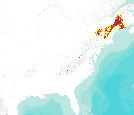This dataset represents actual and predicted suitable habitat for Picea rubens (red spruce, species code 97) in the Eastern United States as measured by importance value based on data obtained from the Forest Inventory and Analysis (FIA) project, current climate conditions, and future climate projections. This summary unit of this dataset is a 20 by 20 kilometer cell. The actual importance value (IV) was calculated based on the number of stems and basal area of a given tree species relative to other tree species on a plot using about 100,000 FIA plots (representing nearly 3 million tree records) in the 37 states within the United States east of the 100th meridian. These importance values were summarized to 20 by...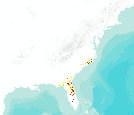This dataset represents actual and predicted suitable habitat for Gordonia lasianthus (loblolly-bay, species code 555) in the Eastern United States as measured by importance value based on data obtained from the Forest Inventory and Analysis (FIA) project, current climate conditions, and future climate projections. This summary unit of this dataset is a 20 by 20 kilometer cell. The actual importance value (IV) was calculated based on the number of stems and basal area of a given tree species relative to other tree species on a plot using about 100,000 FIA plots (representing nearly 3 million tree records) in the 37 states within the United States east of the 100th meridian. These importance values were summarized...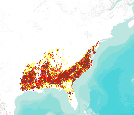This dataset represents actual and predicted suitable habitat for Liquidambar styraciflua (sweetgum, species code 611) in the Eastern United States as measured by importance value based on data obtained from the Forest Inventory and Analysis (FIA) project, current climate conditions, and future climate projections. This summary unit of this dataset is a 20 by 20 kilometer cell. The actual importance value (IV) was calculated based on the number of stems and basal area of a given tree species relative to other tree species on a plot using about 100,000 FIA plots (representing nearly 3 million tree records) in the 37 states within the United States east of the 100th meridian. These importance values were summarized...This dataset represents actual and predicted suitable habitat for Sorbus americana (American mountain-ash, species code 935) in the Eastern United States as measured by importance value based on data obtained from the Forest Inventory and Analysis (FIA) project, current climate conditions, and future climate projections. This summary unit of this dataset is a 20 by 20 kilometer cell. The actual importance value (IV) was calculated based on the number of stems and basal area of a given tree species relative to other tree species on a plot using about 100,000 FIA plots (representing nearly 3 million tree records) in the 37 states within the United States east of the 100th meridian. These importance values were summarized...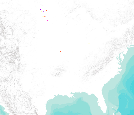This dataset represents actual and predicted suitable habitat for Salix amygdaloides (peachleaf willow, species code 921) in the Eastern United States as measured by importance value based on data obtained from the Forest Inventory and Analysis (FIA) project, current climate conditions, and future climate projections. This summary unit of this dataset is a 20 by 20 kilometer cell. The actual importance value (IV) was calculated based on the number of stems and basal area of a given tree species relative to other tree species on a plot using about 100,000 FIA plots (representing nearly 3 million tree records) in the 37 states within the United States east of the 100th meridian. These importance values were summarized...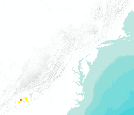This dataset represents actual and predicted suitable habitat for Halesia spp. (silverbell, species code 580) in the Eastern United States as measured by importance value based on data obtained from the Forest Inventory and Analysis (FIA) project, current climate conditions, and future climate projections. This summary unit of this dataset is a 20 by 20 kilometer cell. The actual importance value (IV) was calculated based on the number of stems and basal area of a given tree species relative to other tree species on a plot using about 100,000 FIA plots (representing nearly 3 million tree records) in the 37 states within the United States east of the 100th meridian. These importance values were summarized to 20 by...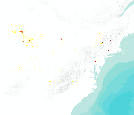This dataset represents actual and predicted suitable habitat for Juglans cinerea (butternut, species code 601) in the Eastern United States as measured by importance value based on data obtained from the Forest Inventory and Analysis (FIA) project, current climate conditions, and future climate projections. This summary unit of this dataset is a 20 by 20 kilometer cell. The actual importance value (IV) was calculated based on the number of stems and basal area of a given tree species relative to other tree species on a plot using about 100,000 FIA plots (representing nearly 3 million tree records) in the 37 states within the United States east of the 100th meridian. These importance values were summarized to 20...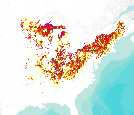This dataset represents actual and predicted suitable habitat for Acer saccharum (sugar maple, species code 318) in the Eastern United States as measured by importance value based on data obtained from the Forest Inventory and Analysis (FIA) project, current climate conditions, and future climate projections. This summary unit of this dataset is a 20 by 20 kilometer cell. The actual importance value (IV) was calculated based on the number of stems and basal area of a given tree species relative to other tree species on a plot using about 100,000 FIA plots (representing nearly 3 million tree records) in the 37 states within the United States east of the 100th meridian. These importance values were summarized to...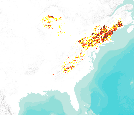This dataset represents actual and predicted suitable habitat for Tsuga canadensis (eastern hemlock, species code 261) in the Eastern United States as measured by importance value based on data obtained from the Forest Inventory and Analysis (FIA) project, current climate conditions, and future climate projections. This summary unit of this dataset is a 20 by 20 kilometer cell. The actual importance value (IV) was calculated based on the number of stems and basal area of a given tree species relative to other tree species on a plot using about 100,000 FIA plots (representing nearly 3 million tree records) in the 37 states within the United States east of the 100th meridian. These importance values were summarized...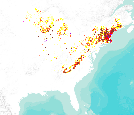This dataset represents actual and predicted suitable habitat for Pinus strobus (eastern white pine, species code 129) in the Eastern United States as measured by importance value based on data obtained from the Forest Inventory and Analysis (FIA) project, current climate conditions, and future climate projections. This summary unit of this dataset is a 20 by 20 kilometer cell. The actual importance value (IV) was calculated based on the number of stems and basal area of a given tree species relative to other tree species on a plot using about 100,000 FIA plots (representing nearly 3 million tree records) in the 37 states within the United States east of the 100th meridian. These importance values were summarized...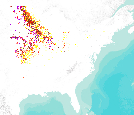This dataset represents actual and predicted suitable habitat for Quercus macrocarpa (bur oak, species code 823) in the Eastern United States as measured by importance value based on data obtained from the Forest Inventory and Analysis (FIA) project, current climate conditions, and future climate projections. This summary unit of this dataset is a 20 by 20 kilometer cell. The actual importance value (IV) was calculated based on the number of stems and basal area of a given tree species relative to other tree species on a plot using about 100,000 FIA plots (representing nearly 3 million tree records) in the 37 states within the United States east of the 100th meridian. These importance values were summarized to 20...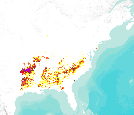This dataset represents actual and predicted suitable habitat for Pinus echinata (shortleaf pine, species code 110) in the Eastern United States as measured by importance value based on data obtained from the Forest Inventory and Analysis (FIA) project, current climate conditions, and future climate projections. This summary unit of this dataset is a 20 by 20 kilometer cell. The actual importance value (IV) was calculated based on the number of stems and basal area of a given tree species relative to other tree species on a plot using about 100,000 FIA plots (representing nearly 3 million tree records) in the 37 states within the United States east of the 100th meridian. These importance values were summarized to...This dataset represents actual and predicted suitable habitat for Ulmus crassifolia (cedar elm, species code 973) in the Eastern Untied States as measured by importance value based on data obtained from the Forest Inventory and Analysis (FIA) project, current climate conditions, and future climate projections. This summary unit of this dataset is a 20 by 20 kilometer cell. The actual importance value (IV) was calculated based on the number of stems and basal area of a given tree species relative to other tree species on a plot using about 100,000 FIA plots (representing nearly 3 million tree records) in the 37 states within the United States east of the 100th meridian. These importance values were summarized to...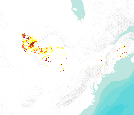This dataset represents actual and predicted suitable habitat for Larix laricina (tamarack (native), species code 71) in the Eastern United States as measured by importance value based on data obtained from the Forest Inventory and Analysis (FIA) project, current climate conditions, and future climate projections. This summary unit of this dataset is a 20 by 20 kilometer cell. The actual importance value (IV) was calculated based on the number of stems and basal area of a given tree species relative to other tree species on a plot using about 100,000 FIA plots (representing nearly 3 million tree records) in the 37 states within the United States east of the 100th meridian. These importance values were summarized...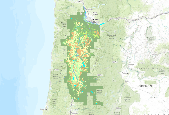This map is a reinterpretation of a more extensive set of polygons demonstrating estimated original (pre 1850) vegetation types for the Willamette Valley. Vegetation type classifications were simplified and recombined for ease of comparison with currently used classifications: i.e. Shrubland, willow swamp was joined with other wetland types, though areas with that original designation can still be found using the "Information" function in ArcMap. The vegetation polygons can be laid over a basemap of current road system for use in comparing original vegetation with current vegetation status, and for locating extant remnants of original plant communities. I believe that the information contained within these...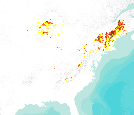This dataset represents actual and predicted suitable habitat for Betula alleghaniensis (yellow birch, species code 371) in the Eastern United States as measured by importance value based on data obtained from the Forest Inventory and Analysis (FIA) project, current climate conditions, and future climate projections. This summary unit of this dataset is a 20 by 20 kilometer cell. The actual importance value (IV) was calculated based on the number of stems and basal area of a given tree species relative to other tree species on a plot using about 100,000 FIA plots (representing nearly 3 million tree records) in the 37 states within the United States east of the 100th meridian. These importance values were summarized...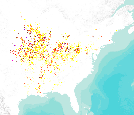This dataset represents actual and predicted suitable habitat for Ulmus rubra (slippery elm, species code 975) in the Eastern United States as measured by importance value based on data obtained from the Forest Inventory and Analysis (FIA) project, current climate conditions, and future climate projections. This summary unit of this dataset is a 20 by 20 kilometer cell. The actual importance value (IV) was calculated based on the number of stems and basal area of a given tree species relative to other tree species on a plot using about 100,000 FIA plots (representing nearly 3 million tree records) in the 37 states within the United States east of the 100th meridian. These importance values were summarized to 20...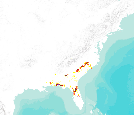This dataset represents actual and predicted suitable habitat for Quercus laevis (turkey oak, species code 819) in the Eastern United States as measured by importance value based on data obtained from the Forest Inventory and Analysis (FIA) project, current climate conditions, and future climate projections. This summary unit of this dataset is a 20 by 20 kilometer cell. The actual importance value (IV) was calculated based on the number of stems and basal area of a given tree species relative to other tree species on a plot using about 100,000 FIA plots (representing nearly 3 million tree records) in the 37 states within the United States east of the 100th meridian. These importance values were summarized to 20...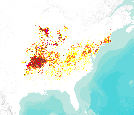This dataset represents actual and predicted suitable habitat for Quercus velutina (black oak, species code 837) in the Eastern United States as measured by importance value based on data obtained from the Forest Inventory and Analysis (FIA) project, current climate conditions, and future climate projections. This summary unit of this dataset is a 20 by 20 kilometer cell. The actual importance value (IV) was calculated based on the number of stems and basal area of a given tree species relative to other tree species on a plot using about 100,000 FIA plots (representing nearly 3 million tree records) in the 37 states within the United States east of the 100th meridian. These importance values were summarized to 20...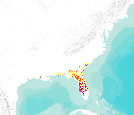This dataset represents actual and predicted suitable habitat for Quercus virginiana (live oak, species code 838) in the Eastern United States as measured by importance value based on data obtained from the Forest Inventory and Analysis (FIA) project, current climate conditions, and future climate projections. This summary unit of this dataset is a 20 by 20 kilometer cell. The actual importance value (IV) was calculated based on the number of stems and basal area of a given tree species relative to other tree species on a plot using about 100,000 FIA plots (representing nearly 3 million tree records) in the 37 states within the United States east of the 100th meridian. These importance values were summarized to... «12345...7»